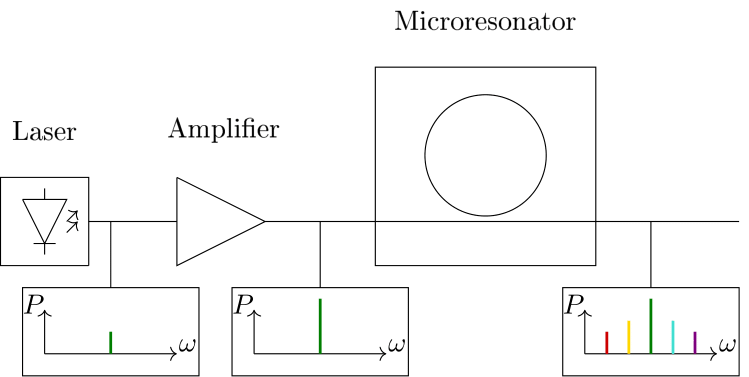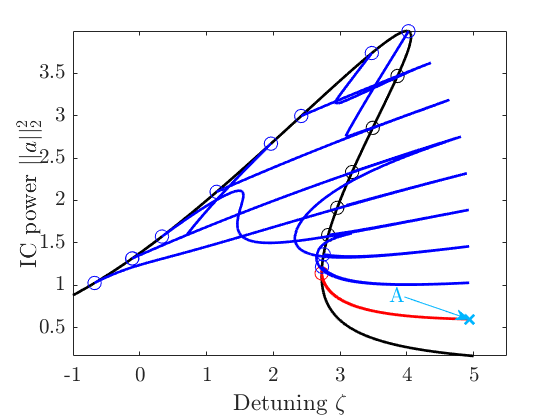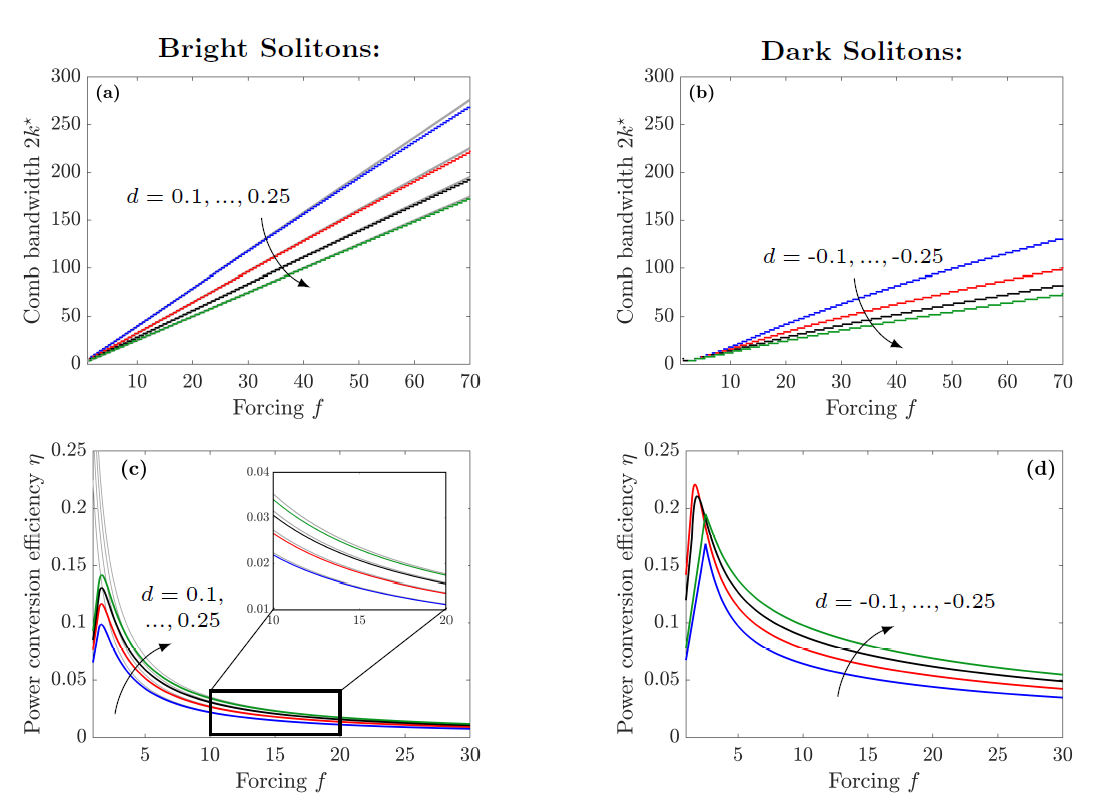# Project B3 • Frequency combs

### Principal investigators

 Prof. Dr. Tobias Jahnke (7/2015 - ) Prof. Dr. Christian Koos (7/2015 - ) Prof. Dr. Wolfgang Reichel (7/2015 - )

### Project summary

A frequency comb is an optical signal consisting of a multitude of equidistantly spaced optical frequencies. Since their discovery by Theodor Hänsch awarded with the Nobel prize in 2005, their tremendous applicability was demonstrated in e.g. optical metrology, frequency metrology and optical communications. In this project, we aim to find a mathematical and electrotechnical framework for the generation of frequency combs with several key characteristics.

Particularly interesting comb sources are nonlinear Kerr microresonators, cf. Figure 1. These chip-scale devices allow for the generation of frequency combs in setups with a footprint comparable to a matchbox, enabling their use in small and mobile objects such as autonomous cars, servers in data-centres and handhelds. In proof-of-principle experiments, we demonstrated the application of microresonator frequency combs in optical communications at data transmission rates of more than 50 Tbits/s through a single optical fiber, [ECOC16, Nature17]. Furthermore, we performed optical distance measurements and achieved a sub-µm resolution at record sampling rates of 100 MHz [CLEO17, Science18].Figure 1. Schematic of system for frequency comb generation. A tunable continuous wave (cw) laser emits light at a frequency $\\omega$, which is amplified by an optical amplifier. Then, the light is coupled into a microresonator on a microchip. By tuning the frequency of the laser, a frequency comb emerges in the microresonator, which can then be coupled out of the chip again for further use.

To enable this technology for a widespread use, key characteristics such as energy efficiency and potential for mass production need to be investigated. We address these challenges in an interdisciplinary team consisting of mathematicians and engineers by phrasing practical demands and answering these with mathematical techniques. We focus on the optical power conversion efficiency of a driving optical field inside the resonator and the emerging frequency comb. Furthermore, we explore the potential of different material platforms. Here, especially Silicon is of interest due to its widespread use in the semiconductor industry.

From the mathematical point of view, the field inside the resonator is approximated by a complex-valued function $a(t,x)$, which depends on time $t$ and intracavity position $x$, and satisfies the Lugiato–Lefever–equation (LLE) $\\frac{\\partial a(t,x)}{\\partial t}=\left[-1-\\text{i}\\zeta+\\text{i}d\\frac{\\partial^2}{\\partial x^2}+\\text{i}|a(t,x)|^2\\right]a(t,x)+f, \\quad a(t,x+2\\pi)=a(t,x)$ The LLE is a damped and forced nonlinear Schrödinger equation with parameters given by forcing $f$ (amplitude of the driving field), detuning $\\zeta$ (relative offset between frequency of the driving field and a resonance frequency of the microresonator) and second-order dispersion $d$. When pumping the microresonator with an incoming single-frequency field, the Kerr-nonlinear term in the LLE gives rise to new spectral components, resulting in a frequency comb. Of most interest are soliton frequency comb states. These are stationary strongly localized solutions in space, which feature a broad spectrum in the frequency domain, cf. Figure 2.Figure 2. Soliton solution and corresponding frequency comb for $d=0.01,\\,\\zeta=80$ and $f=23$.

In order to find soliton states analytically, several approaches for the stationary equation were used. The first approach is continuation for $d>0$. We consider a reformulated version of the equation $-du''+(\\zeta-\\epsilon\\text{i})u-|u|^2u+\\text{i}f=0$ on the real line. For $\\epsilon=f=0$ the soliton solution can be calculated explicitly, and then analytically continued into the regime where $\\epsilon>0$ and $f>0$. This approach can also be used numerically as these solutions serve as approximations on a bounded interval. Scaling them back leads to a map of parameters where we can expect soliton solutions.

The second approach addresses bifurcation with respect to the parameter $\\zeta$, cf. [MR17], and preprint [ECOC16]. At certain values, non-trivial solutions bifurcate from the trivial curve, cf. Figure 3.Figure 3. Bifurcation diagrams for $d=0.1$ and $f=2$ (left) and for $d=-0.1$ and $f=2$ (right).

In our investigations, we observe the following heuristic:
For $d>0$ the most localized solitons are at the first turning point of the last bifurcating branch (Label A).
For $d<0$ the most localized solitons are at the second turning point of the first bifurcating branch (Label B).
Using this heuristic, we can identify parameter regimes where the most localized soliton solutions are found. Defining quality measures such as combwidth and power conversion efficiency, this enables us to determine a universal conversion efficiency map, cf. Figure 4, which is essential for designing microresonators embedded in integrated photonic systems, cf. [GTMKJR19].Figure 4. Combwidth and power conversion efficiency for $d>0$ and $d<0$.

Within our project we also consider numerical time integration of the LLE via a Strang-Splitting between its nonlinear and dispersive part; cf. [JMS17]. One of our observation was that time integration follow the bifurcation branches when the detuning is swept over time.

Recently ([GMR18]), we started to discuss the effects of two photon absorption and free carrier absorption, which play an important role when considering, e.g., silicon based mircoresonator devices. Kerr frequency combs are amazing objects, with great applications and wonderful mathematical features. A lot is still to be discovered.

## Publications

1. , , , , , and . Bandwidth and conversion efficiency analysis of dissipative Kerr soliton frequency combs based on bifurcation theory. Phys. Rev. A, 100(3):033819, September . [preprint] [bibtex]

2. . Global secondary bifurcation, symmetry breaking and period-doubling. Topol. Methods Nonlinear Anal., 53(2):779–800, June . [preprint] [bibtex]

3. , , , , , , , , , , , , and . Ultrafast optical ranging using microresonator soliton frequency combs. Science, 359(6378):887–891, February . [bibtex]

4. , , and . Strang splitting for a semilinear Schrödinger equation with damping and forcing. J. Math. Anal. Appl., 455(2):1051–1071, November . [preprint] [bibtex]

5. , , , , , , , , , , , , , , and . Microresonator-based solitons for massively parallel coherent optical communications. Nature, 546:274–279, June . [bibtex]

6. , , , , , , , , , , , and . Ultrafast dual-comb distance metrology using dissipative Kerr solitons. In Conference on Lasers and Electro-Optics, STh4L.6, May . Optical Society of America. [bibtex]

7. and . A priori bounds and global bifurcation results for frequency combs modeled by the Lugiato-Lefever equation. SIAM J. Appl. Math., 77(1):315–345, February . [preprint] [bibtex]

8. , , , , , , , , , and . 34.6 Tbit/s WDM transmission using soliton Kerr frequency combs as optical source and local oscillator. In European Conference on Optical Communication (ECOC), pages 415–417, December . [bibtex]

9. , , , , , , , , , , , , , and . 50 Tbit/s massively parallel WDM transmission in C and L band using interleaved cavity-soliton Kerr combs. In Conference on Lasers and Electro-Optics, STu1G.1, June . [preprint] [bibtex]

## Preprints

1. , , and . The Lugiato–Lefever equation with nonlinear damping caused by two photon absorption. CRC 1173 Preprint 2018/44, Karlsruhe Institute of Technology, November . [bibtex]

2. and . Stochastic Galerkin-collocation splitting for PDEs with random parameters. CRC 1173 Preprint 2018/28, Karlsruhe Institute of Technology, October . [bibtex]

## Theses

1. . Continuation and bifurcation of frequency combs modeled by the Lugiato–Lefever equation. PhD thesis, Karlsruhe Institute of Technology (KIT), February . [bibtex]

2. . Terabit-rate transmission using optical frequency comb sources. PhD thesis, Karlsruhe Institute of Technology (KIT), July . [bibtex]

Former staff
Name Title Function
Dipl.-Phys. Doctoral researcher
Dr. Doctoral researcher
M.Sc. Doctoral researcher